﻿ 液体静压电主轴轴心轨迹影响因素研究

# 液体静压电主轴轴心轨迹影响因素研究Study on the Evolution Law of Spindle Trajectories of Hydrostatic Motorized Spindle under Cutting Load

Abstract: With the development of high-speed machining technology, sliding bearings are more and more widely used in high-speed machining. Sliding bearings and motorized spindles are directly involved in high-speed machining. Their dynamic performance determines the processing efficiency and quality of high-speed machine tools. Aiming at the unbalanced force of the spindle and external disturbance in the process of high-speed machining, knives are generated. Vibration affects the quality of workpiece. Based on Reynolds equation, flow continuity equation and numerical method, the oil film force of hydrostatic bearing is solved to establish the dynamic model of spindle ROTOR-SLIDING bearing. Then the spindle axis trajectory is solved by Euler method, and the influence of spindle speed and milling cutter blade number on the axis trajectory is analyzed. The systematic analysis method of vertical ROTOR-SLIDING bearing provides a practical and reliable basis for optimizing the structure design of motorized spindle and improving the quality and effi-ciency of high-speed machining.

1. 引言

2. 液体静压电主轴轴承–转子动力学模型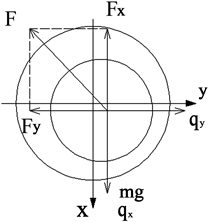Figure 1. Stress model of high speed motorized spindle rotor

$\left\{\begin{array}{l}m\stackrel{¨}{x}={F}_{x}+m{e}_{g}{\omega }_{0}^{2}\mathrm{cos}{\omega }_{0}t+mg\\ m\stackrel{¨}{y}={F}_{y}+m{e}_{g}{\omega }_{0}^{2}\mathrm{sin}{\omega }_{0}t\end{array}$ (1)

$\left\{\begin{array}{l}\stackrel{¨}{X}=\frac{{F}_{x}}{mc{\omega }_{0}^{2}}+\frac{{e}_{g}}{c}\mathrm{cos}\tau +\frac{g}{c{\omega }_{0}^{2}}\\ \stackrel{¨}{Y}=\frac{{F}_{y}}{mc{\omega }_{0}^{2}}+\frac{{e}_{g}}{c}\mathrm{sin}\tau \end{array}$ (2)

3. 液体静压轴承油膜力计算模型的建立与求解

3.1. 液体静压轴承雷诺方程的建立

$\frac{\partial }{\partial x}\left(\frac{{h}^{3}}{\mu }\frac{\partial p}{\partial x}\right)+\frac{\partial }{\partial z}\left(\frac{{h}^{3}}{\mu }\frac{\partial p}{\partial z}\right)=6\frac{\partial \left(Uh\right)}{\partial x}+12\frac{\partial h}{\partial t}$ (3)

z——轴承的轴向坐标(m)；

h——油膜厚度(m)；

U——轴颈的转速(rpm)；

$\mu$ ——润滑油动力粘度(N s/m2)。

$h=c+x\mathrm{cos}\phi +y\mathrm{sin}\phi$ (4)

$\begin{array}{l}x=r\varphi ,\text{\hspace{0.17em}}z=L\lambda ,\text{\hspace{0.17em}}h=cH,\text{\hspace{0.17em}}U=r{\omega }_{0}\\ p=P{P}_{J0},\text{\hspace{0.17em}}t=\tau /\left({\omega }_{0}\right),\text{\hspace{0.17em}}{P}_{J0}=\frac{{r}^{2}{\omega }_{0}\mu }{{c}^{2}}\end{array}$

$\frac{\partial }{\partial \phi }\left({H}^{3}\frac{\partial P}{\partial \phi }\right)+\frac{{r}^{2}}{{l}^{2}}\frac{\partial }{\partial \lambda }\left({H}^{3}\frac{\partial p}{\partial \lambda }\right)=6\frac{\partial H}{\partial \phi }+12\left(\stackrel{˙}{X}\mathrm{cos}\phi +\stackrel{˙}{Y}\mathrm{sin}\theta \right)$ (5)

L——轴承的长度(m)。

3.2. 液体静压轴承流量平衡方程Figure 2. Oil film force calculation diagram

${q}_{in}=\frac{\alpha \text{π}{d}_{0}^{2}}{4}\sqrt{\frac{2\left({p}_{s}-{p}_{i}\right)}{\rho }}$ (6)

${p}_{i}$ ——第i个油腔的油腔压力；

$\alpha$ ——节流系数；

${d}_{0}$ ——小孔节流器孔径；

$\rho$ ——润滑油密度。

${q}_{out}={\int }_{s1+s3}\left(\frac{Uh}{2}-\frac{1}{12}\frac{{h}^{3}}{\mu }\frac{\partial p}{\partial x}\right)\text{d}z+{\int }_{s2+s4}\left(-\frac{1}{12}\frac{{h}^{3}}{\mu }\frac{\partial p}{\partial z}\right)\text{d}x$ (7)

$\begin{array}{r}\frac{\alpha \text{π}{d}_{0}^{2}}{4{\omega }_{0}{c}^{2}L}\sqrt{\frac{2{\omega }_{0}\mu }{\rho }\left({P}_{s}-{P}_{i}\right)}={\int }_{s1+s3}\left(\frac{H}{2}-\frac{1}{12}{H}^{3}\frac{\partial p}{\partial \phi }\right)\text{d}\lambda \\ +{\int }_{s2+s4}\left(-\frac{1}{12}\frac{{r}^{2}}{{L}^{2}}{H}^{3}\frac{\partial p}{\partial \lambda }\right)\text{d}\phi \end{array}$ (8)

3.3. 液体静压轴承油膜力计算

$\begin{array}{c}{F}_{x}={\int }_{0}^{x}{\int }_{0}^{z}p\mathrm{cos}\theta \text{d}x\text{d}z={\int }_{0}^{\text{2π}}{\int }_{0}^{L}{P}_{J0}rLp\mathrm{cos}\theta \text{d}\phi \text{d}\lambda \\ =\eta Lr{\omega }_{0}{\left(\frac{r}{c}\right)}^{2}{\int }_{0}^{\text{2π}}{\int }_{0}^{1}p\mathrm{cos}\theta \text{d}\phi \text{d}\lambda ={F}_{0}\stackrel{¯}{{F}_{x}}\end{array}$ (9)

$\begin{array}{c}{F}_{y}={\int }_{0}^{x}{\int }_{0}^{z}p\mathrm{sin}\theta \text{d}x\text{d}z={\int }_{0}^{\text{2π}}{\int }_{0}^{L}{P}_{J0}rLp\mathrm{sin}\theta \text{d}\phi \text{d}\lambda \\ =\eta Lr{\omega }_{0}{\left(\frac{r}{c}\right)}^{2}{\int }_{0}^{\text{2π}}{\int }_{0}^{1}p\mathrm{cos}\theta \text{d}\phi \text{d}\lambda ={F}_{0}\stackrel{¯}{{F}_{y}}\end{array}$ (10)

$\stackrel{¯}{{F}_{x}}={\int }_{0}^{\text{2π}}{\int }_{0}^{1}p\mathrm{cos}\theta \text{d}\phi \text{d}\lambda =\underset{1}{\overset{m}{\sum }}\underset{1}{\overset{n}{\sum }}{p}_{i,j}\mathrm{cos}{\phi }_{i,j}\Delta \phi \Delta \lambda$ (11)

$\stackrel{¯}{{F}_{y}}={\int }_{0}^{\text{2π}}{\int }_{0}^{1}p\mathrm{sin}\theta \text{d}\phi \text{d}\lambda =\underset{1}{\overset{m}{\sum }}\underset{1}{\overset{n}{\sum }}{p}_{i,j}\mathrm{sin}{\phi }_{i,j}\Delta \phi \Delta \lambda$ (12)

4. 主轴轴心轨迹影响因素仿真分析

4.1. 轴心非线性轨迹模型的建立

$\left\{\begin{array}{l}\stackrel{˙}{X}\left(\tau +\Delta \tau \right)=\stackrel{˙}{X}\left(\tau \right)+\stackrel{¨}{X}\left(\tau +\Delta \tau \right)\Delta \tau \\ X\left(\tau +\Delta \tau \right)=X\left(\tau \right)+\stackrel{˙}{X}\left(\tau +\Delta \tau \right)\Delta \tau \\ \stackrel{˙}{Y}\left(\tau +\Delta \tau \right)=\stackrel{˙}{Y}\left(\tau \right)+\stackrel{¨}{Y}\left(\tau +\Delta \tau \right)\Delta \tau \\ Y\left(\tau +\Delta \tau \right)=Y\left(\tau \right)+\stackrel{˙}{Y}\left(\tau +\Delta \tau \right)\Delta \tau \end{array}$ (13)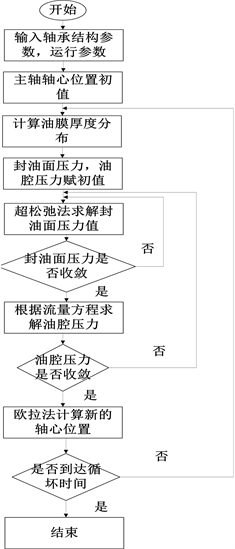Figure 3. Block diagram

4.2. 结构参数对主轴轴心轨迹的影响分析

4.2.1. 半径间隙对主轴轴心轨迹的影响Table 1. Hydrostatic bearing parameters(a) $c=4.5×{10}^{-5}$ 轴心轨迹图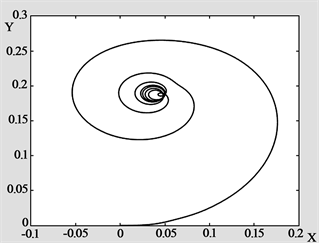(b) $c=5.5×{10}^{-5}$ 轴心轨迹图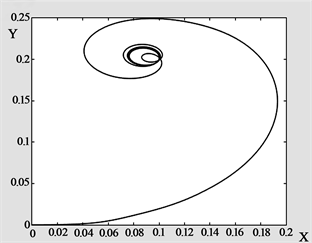(c) $c=6×{10}^{-5}$ 轴心轨迹图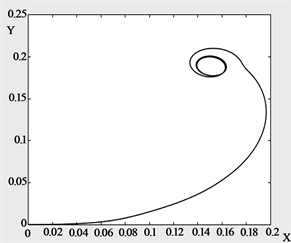(d) $c=6.5×{10}^{-5}$ 轴心轨迹图

Figure 4. The variation of the center ${\epsilon }_{g}$ of the axis trajectory with time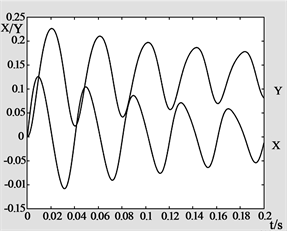(a) $c=4.5×{10}^{-5}$ 轴心X、Y位移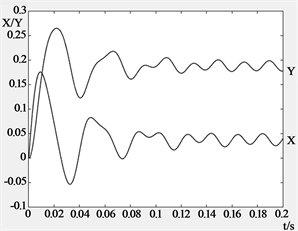(b) $c=5.5×{10}^{-5}$ 轴心X、Y位移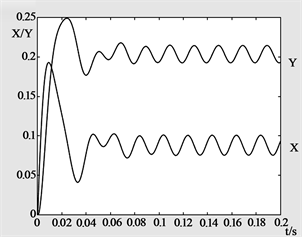(c) $c=6×{10}^{-5}$ 轴心X、Y位移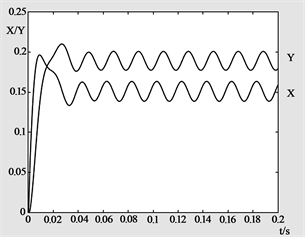(d) $c=6.5×{10}^{-5}$ 轴心X、Y位移

Figure 5. Axis center trajectory X, Y displacement change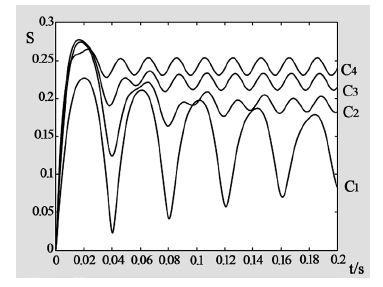Figure 6. Spindle axis radial displacement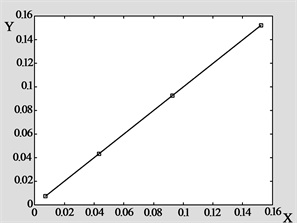Figure 7. Center coordinates of axis trajectory

4.2.2. 轴承的宽径比对轴心轨迹的影响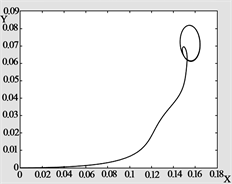(a) $\text{B}/\text{D}=\text{0}\text{.5}$ 轴心轨迹图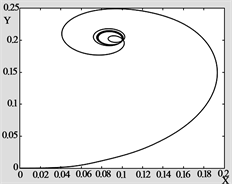(b) $\text{B}/\text{D}=\text{0}\text{.7}$ 轴心轨迹图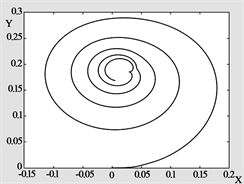(c) $\text{B}/\text{D}=\text{0}\text{.8}$ 轴心轨迹图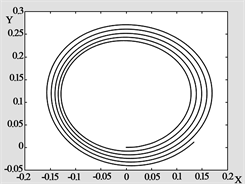(d) $\text{B}/\text{D}=\text{1}\text{.0}$ 轴心轨迹图

Figure 8. Locus of axes with different aspect ratios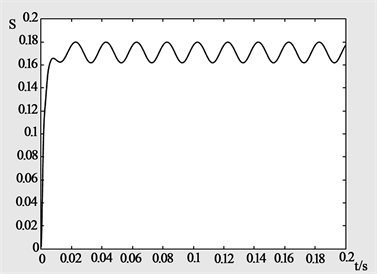(a) $\text{B}/\text{D}=\text{0}\text{.5}$ 主轴轴心径向位移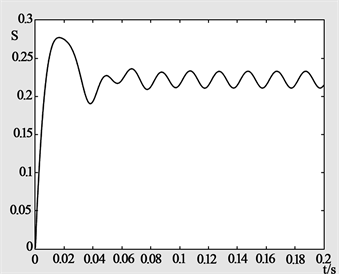(b) $\text{B}/\text{D}=\text{0}\text{.7}$ 主轴轴心径向位移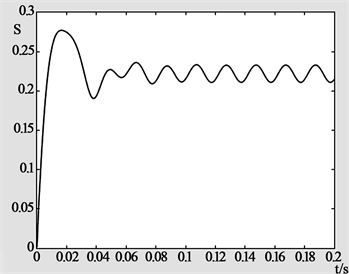(c) $\text{B}/\text{D}=\text{0}\text{.8}$ 主轴轴心径向位移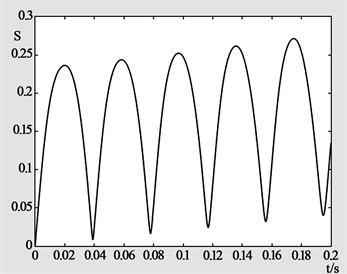(d) $\text{B}/\text{D}=\text{1}\text{.0}$ 主轴轴心径向位移

Figure 9. Spindle axis radial displacement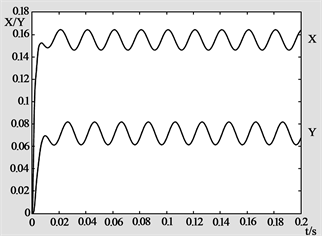(a) $\text{B}/\text{D}=\text{0}\text{.5}$ 轴心X、Y位移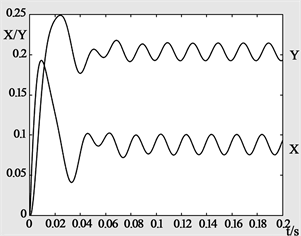(b) $\text{B}/\text{D}=\text{0}\text{.7}$ 轴心X、Y位移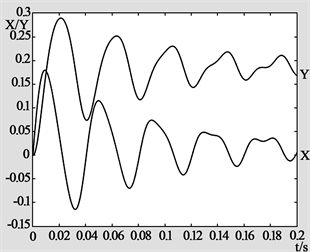(c) $\text{B}/\text{D}=\text{0}\text{.8}$ 轴心X、Y位移(d) $\text{B}/\text{D}=\text{1}\text{.0}$ 轴心X、Y位移

Figure 10. Variation of axis X、Y displacement under different B/D

5. 结论

1) 本文采用有限差分法求解液体静压轴承油膜力，再采用欧拉法求解主轴轴心非线性轨迹。结果表明，液体静压电主轴轴心将不断的做收敛运动直至稳定在平衡位置，从而表明了液体静压电主轴系统具有一定稳定性。

2) 本文在液体静压电主轴轴心轨迹模型上，分析了轴承的间隙对轴心轨迹规律的影响；结果表明，静压轴承宽径比(B/D)对轴心轨迹性能有着重要影响，当轴承宽径比(B/D)增加，主轴轴心的收敛速度降低，系统的稳定性下降，但其等效刚度逐渐增加。

3) 本文采用单变量控制法，进一步分析了静压轴承宽径比(B/D)对轴心轨迹性能有着重要影响，结果表明，当轴承宽径比(B/D)增加，主轴轴心的收敛速度降低，系统的稳定性下降，但其等效刚度逐渐增加。因此合理选取静压轴承的宽径比(B/D)可以得出最优的轴心轨迹性能。

1) 参与研究国家自然科学基金“多场耦合下液体静压电主轴轴心轨迹的动态演化机理与主动控制研究”(项目编号：51875198)。

2) 参与研究湖南省自然科学基金“磁悬浮电主轴轴心轨迹的主动控制理论及方法研究”(项目编号：2017JJ2090)。

3) 参与研究教育厅基金“高速电主轴的电磁解耦方法与-转子刀具系统输出动力学行为之间的关系研究”(项目编号：12B042)。

 马金奎. 滑动轴承非线性轴心轨迹的瞬态与周期特性研究[D]: [博士学位论文]. 济南: 山东大学, 2010.

 Hattori, H. (2008) Dynamic Analysis of a Rotor-Journal Bearing System with Large Dynamic Loads. Stiffness and Damping Coeffcient Variation in Bearing Oil Films. JSME International Journal, 36, 251-257.

 Ramesh, J., Majumdar, B.C. and Rao, N.S. (1997) Stability Cha-racteristics of Rough Submerged Oil Elliptical Bearings under Dynamic Load. Tribology International, 30, 857-863.
https://doi.org/10.1016/S0301-679X(97)00067-4

 Ene, N.M. and Dimofte Jr., F.T.G.K. (2007) A Stability Analysis for a Hydrodynamic Three-Wave Journal Bearing. Tribology International, 41, 434-442.
https://doi.org/10.1016/j.triboint.2007.10.002

 Sinhasana, R. and Goyalb, K.C. (1995) Transient Response of a Two-Lobe Journal Bearing Lubricated with Non- Newtonian Lubricant. Tribology International, 28, 233-239.
https://doi.org/10.1016/0301-679X(95)00007-Q

 Meruane, V. and Pascual, R. (2008) Identification of Nonlinear Dynamic Coefficients in Plain Journal Bearings. Tribology International, 41, 743-754.
https://doi.org/10.1016/j.triboint.2008.01.002

 Zhou, H., Zhao, S., Xu, H., et al. (2004) An Experimental Study on Oil-Film Dynamic Coefficients. Tribology International, 37, 245-253.
https://doi.org/10.1016/j.triboint.2003.08.002

 张洪, 李广明, 孟凡明. 分形参数对轴心轨迹的影响[J]. 润滑与密封, 2006(6): 118-120.

 冯凯, 张优云. 低气压对发动机轴承性能的影响[J]. 润滑与密封, 2007, 32(3): 12-17.

 朱汉华, 严新平, 刘正林, 等. 冲击载荷下船舶轴系转速与回旋振动间影响研究[J]. 武汉理工大学学报(交通科学与工程版), 2008, 32(6): 983-985.

 Tiwari, R. and Chakravarthy, V. (2006) Simultaneous Identi-fication of Residual Unbalances and Bearing Dynamic Parameters from Impulse Responses of Rotor-Bearing Systems. Mechanical Systems & Signal Processing, 20, 1590-1614.
https://doi.org/10.1016/j.ymssp.2006.01.005

 何芝仙, 桂长林, 李震, 等. 冲击载荷作用下计入轴倾斜的轴–轴承系统动力学摩擦学行为研究[J]. 轴承, 2007(3): 17-21.

 Rho, B.H. and Kim, K.W. (2003) Acoustical Properties of Hydrodynamic Journal Bearings. Tribology International, 36, 61-66.
https://doi.org/10.1016/S0301-679X(02)00132-9

Top